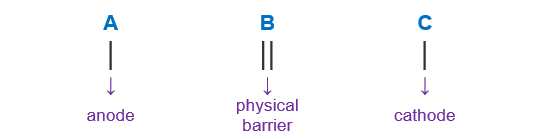# Problem: A student devises an electrochemical cell with the following cell notation:Cr (s) | Cr2+ (aq, 0.02 M) | | Co2+ (aq, 0.9 M) | Co (s)a) Write the half-cell reactions and the overall reaction for this galvanic cell.

###### FREE Expert Solution

We’re being asked to write the half-cell reactions and the overall reaction for this galvanic cell:

Cr (s) | Cr2+ (aq, 0.02 M) | | Co2+ (aq, 0.9 M) | Co (s)

When writing a cell notation, we use the following format – “as easy as ABCLoss of Electron is an Oxidation process undergone by the Reducing Agent (LEORA). In the Anode, Oxidation happens (An Ox)

Gain of Electron is a Reduction process undergone by the Oxidizing Agent (GEROA). Reduction happens in the Cathode (Red Cat)

We can determine the anode and cathode by comparing their E° values.

• ↓ E° → oxidation → anode

• ↑ E° → reduction → cathode

83% (105 ratings)###### Problem Details

A student devises an electrochemical cell with the following cell notation:

Cr (s) | Cr2+ (aq, 0.02 M) | | Co2+ (aq, 0.9 M) | Co (s)

a) Write the half-cell reactions and the overall reaction for this galvanic cell.